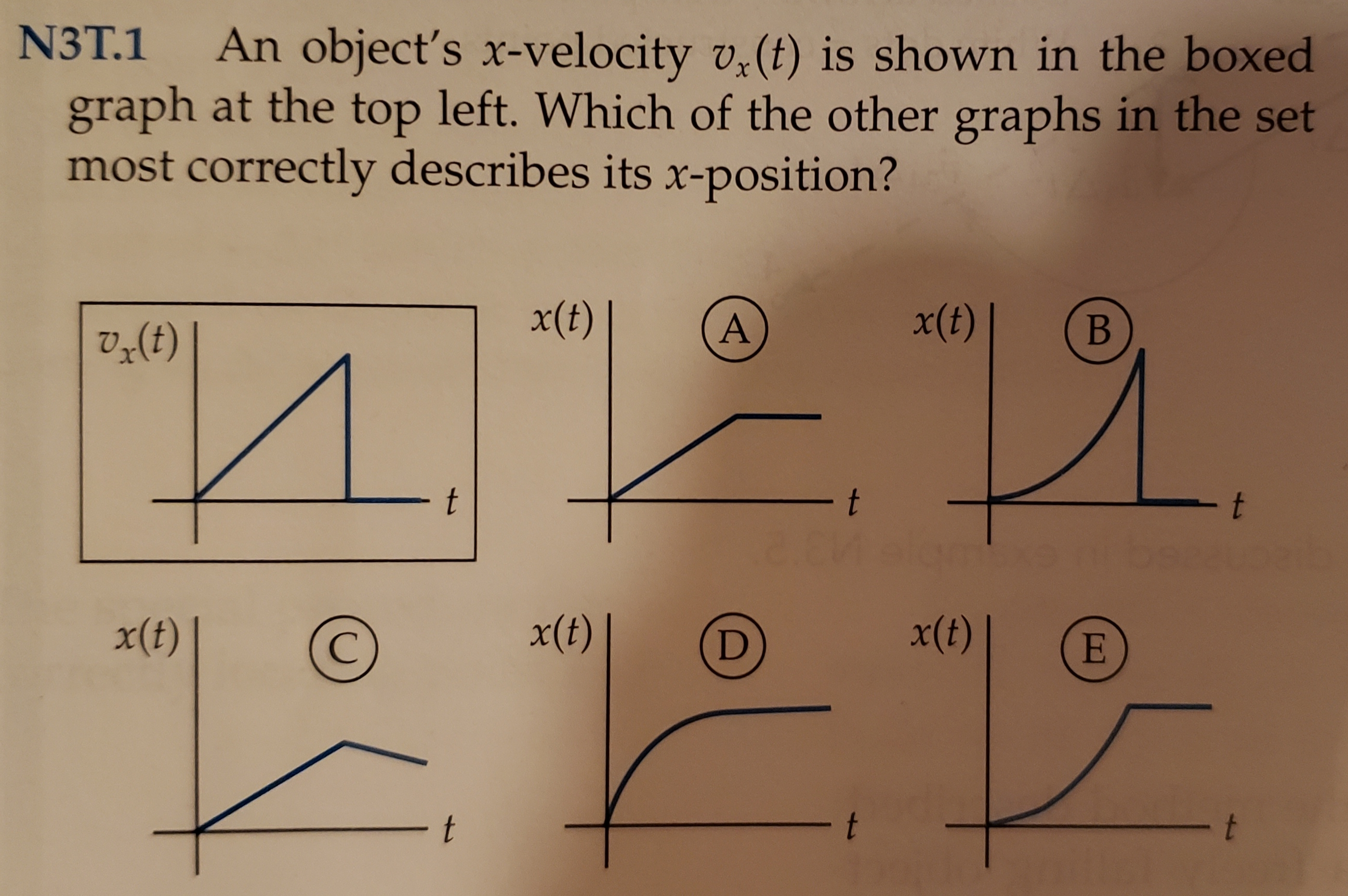# N3T.1An object's x-velocity v,(t) is shown in the boxedgraph at the top left. Which of the other graphs in the setmost correctly describes its x-position?x(t)x(t)ВИ.12tttx(t)x(t)x(t)Et

Question
19 viewshelp_outlineImage TranscriptioncloseN3T.1 An object's x-velocity v,(t) is shown in the boxed graph at the top left. Which of the other graphs in the set most correctly describes its x-position? x(t) x(t) В И. 12 t t t x(t) x(t) x(t) E t fullscreen
check_circle

Step 1

Now, consider the velocity verses time diagram. In the diagram complete motion is in two part, the first part is velocity is increasing and the in the second part the velocity becomes zero.

Step 2

For the first part of the motion.

Using the concept of straight line. The equation of velocity is:

Step 3

Use the definition of velocity and rewri...

### Want to see the full answer?

See Solution

#### Want to see this answer and more?

Solutions are written by subject experts who are available 24/7. Questions are typically answered within 1 hour.*

See Solution
*Response times may vary by subject and question.
Tagged in

### Kinematics